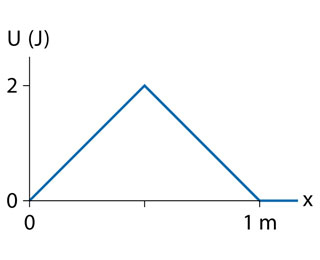# Problem: shows the potential energy of a system in which a particle moves along the x-axis.Draw a graph of the force Fx as a function of position x for the x(0,0.5).Draw a graph of the force Fx as a function of position x for the x(0.5,1).

96% (17 ratings)
###### Problem Detailsshows the potential energy of a system in which a particle moves along the x-axis.

Draw a graph of the force Fx as a function of position x for the x(0,0.5).

Draw a graph of the force Fx as a function of position x for the x(0.5,1).